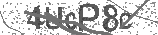﻿ The Order of Operations Song (PEMDAS) | Silly School Songs | Safe Videos for Kids
Welcome

# The Order of Operations Song (PEMDAS) | Silly School Songs

Thanks! Share it with your friends!

URL

You disliked this video. Thanks for the feedback!

Sorry, only registred users can create playlists.URLFind Related Videos  added
41 Views## Description

Learn the Order of Operations (including an important note about PEMDAS) with this fun, funky and very catchy tune. For more information and music, visit http://sillyschoolsongs.com.

Lyrics:

Parenthesis, Exponents
Multiply or Divide, Add or Subtract
Parenthesis, Exponents
Multiply or Divide, Add or Subtract
It's the order of operations
The order of operations
Parenthesis, Exponents
Multiply or Divide, Add or Subtract

Let's talk about the Order of Operations
Keep in mind as you listen to this verse
That the rules are designed to tell you what comes first
(Step One!)
When you see parenthesis jump right in
It's the place that you always begin
(Step Two!)
Keep in mind exponents are next
Multiply those out, you got two steps left
(Step Three!)
Then you multiply or divide
Make sure you always move from left to right
(Step Four!)
Keep it moving with the left-to-right action

Parenthesis, Exponents
Multiply or Divide, Add or Subtract
Parenthesis, Exponents
Multiply or Divide, Add or Subtract
It's the order of operations
The order of operations
Parenthesis, Exponents
Multiply or Divide, Add or Subtract

You may have heard about PEMDAS
It's a good acronym, but not so fast
It makes it seem like you always have to multiply first and
Divide last, but wait a minute class!
You always go in the order they appear
From left to right, just to make things clear
Same thing is true with addition and subtraction
Always be sure to pay attention to direction!

Parenthesis, Exponents
Multiply or Divide, Add or Subtract
Parenthesis, Exponents
Multiply or Divide, Add or Subtract
It's the order of operations
The order of operations
Parenthesis, Exponents
Multiply or Divide, Add or SubtractBe the first to comment